# OFFSET Function

Returns a specified number of rows and columns from a specified range

## What is the OFFSET Function?

The OFFSET function is categorized under Excel Lookup and Reference functions. OFFSET will return a range of cells. That is, it will return a specified number of rows and columns from an initial range that was specified.

In financial analysis, we often use Pivot Tables and Charts. The OFFSET function can be used to build a dynamic named range for pivot tables or charts to make sure that the source data is always up to date.

### Formula

= OFFSET(reference, rows, cols, [height], [width])

The OFFSET function uses the following arguments:

1. Reference (required argument) – This is the cell range that is to be offset. It can be either single cell or multiple cells
2. Rows (required argument) – This is the number of rows from the start (upper left) of the supplied reference, to the start of the returned range.
3. Cols (required argument) – The number of columns from the start (upper left) of the supplied reference, to the start of the returned range.
4. Height (optional argument) – Specifies the height of the returned range. If omitted, the returned range is the same height as the supplied reference argument.
5. Width (optional argument) – This specifies the width of the returned range. If omitted, the returned range is the same width as the supplied reference.

### How to use the OFFSET Function in Excel?

As a worksheet function, the OFFSET function can be entered as part of a formula in a cell of a worksheet. To understand the uses of the function, let us consider a few examples:

#### Example 1

Let’s say we are given the weekly earnings for 5 weeks below: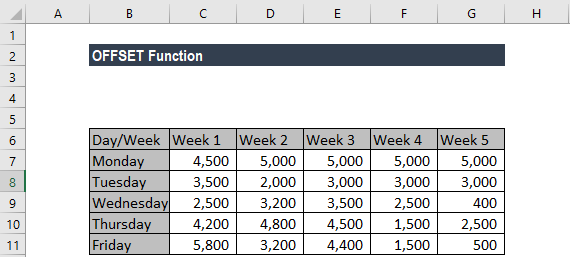Now, if we insert the formula =OFFSET(A3,3,1) in cell B1, it will give us the value 2,500, which is 3 rows down on column right, as shown below: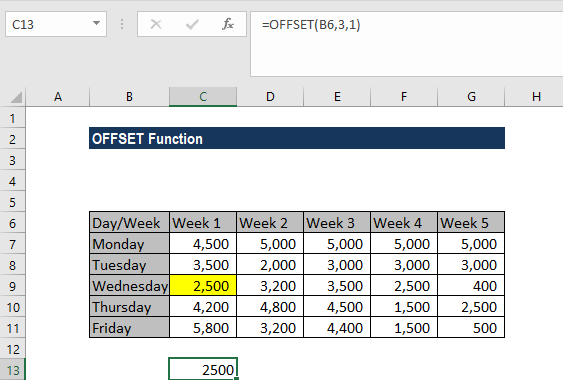In the above example, as the height and width of the returned range are same as the reference range, we omitted the reference range.

#### Example 2

Continuing with the same example, suppose we want the earnings for Thursday for all the weeks. In this scenario, we will use the formula {=OFFSET(B7,3,1,1,5)}.

We get the results below: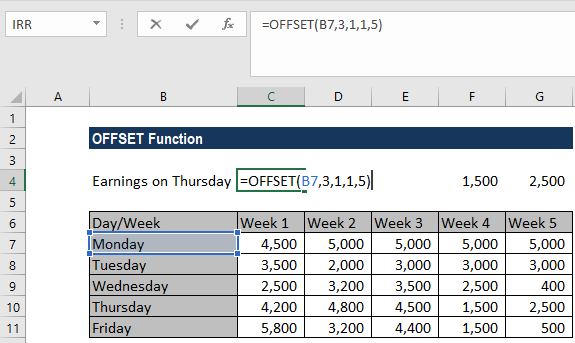In the example above:

1. As the results of the OFFSET function are to occupy more than one cell, it is necessary to enter the function as an array. It can be seen by the parentheses that surround the formula in the Formula bar.
2. In the given formula, as the width of the returned range is more than the width of the reference range, we specified the height and width arguments.

#### Example 3

Continuing with the same example, suppose we want the sum total of the earnings for Week 3. We use the formula =SUM(OFFSET(G6,1,-2,5)).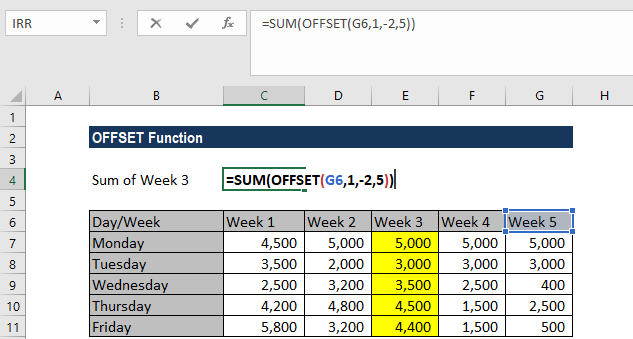We get the results below: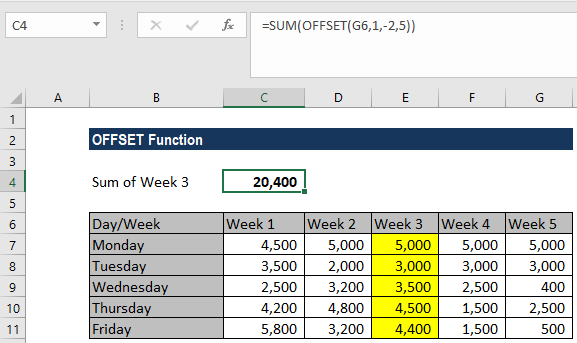In the example above:

1. The array of values returned by the OFFSET function is directly entered into the SUM function, which returns a single value. Hence, we do not need it to enter as an array formula.
2. The height of the offset range is greater than the height of the reference range and so the [height] argument is entered as the value 5.
3. The width of the offset range is the same as the width of the reference range and so the [width] argument was omitted from the function.

### A few notes about the OFFSET Function:

1. #REF! error – Occurs when the range resulting from the requested offset is invalid. For example, it extends beyond the edge of the worksheet.
2. #VALUE! error – Occurs if any of the supplied rows, cols, [height] or [width] arguments is non-numeric.

Thanks for reading CFI’s guide to important Excel functions! By taking the time to learn and master these functions, you’ll significantly speed up your financial modeling. To learn more, check out these additional CFI resources:

• Excel Functions for Finance